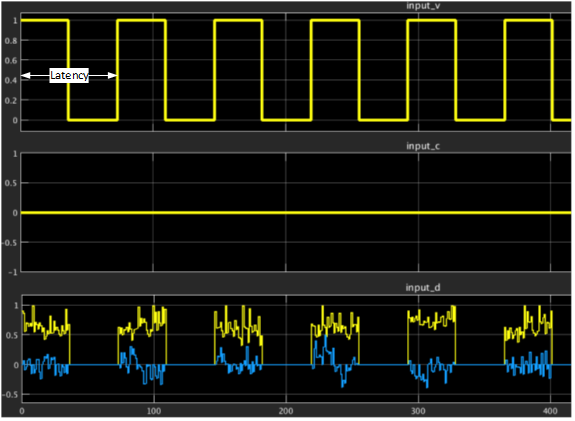ID 683337
Date 3/23/2022
Public

## 6.13.13. Cholesky-based Matrix Inversion

Matrix inversion has many applications in wireless communications, e.g. digital predistortion (DPD) for RF linearization and multiple-input multiple-output (MIMO) detection. Matrix inversion algorithms typically require high-resolution numerics to guarantee accuracy and numerical stability. The implementation is normally resource demanding in particular if the matrix dimension grows. The DSP Builder Cholesky-based Matrix Inversion reference design offers an efficient implementation of matrix inversion for minimized resource utilization and improved latency and throughput. The Cholesky decomposition technique inverts a positive-definite real or complex square matrix. Cholesky decomposition-based matrix inversion is more efficient than direct matrix inversion.
Figure 69. Matrix inversion based on Cholesky decompositionThe figure shows the three steps of implementing a Hermitian matrix inversion using Cholesky decomposition:
1. Cholesky decomposition
2. Triangular matrix inversion through forward substitution
3. Triangular matrix multiplication

The Cholesky decomposition calculates the reciprocal values of the diagonal elements of L, which the triangular matrix inversion requires. The design propagates those values to the output interface of the Cholesky decomposition reducing resource usage and latency.

Assuming matrix A is an NxN positive-definite square matrix, Cholesky decomposition of A into lower and upper triangular matrices, L, and L H is given by:

A = LH

The inverse of Hermitian A, A -1 is:

The design performs Cholesky decomposition and calculates the inverse of L, , through forward substitution. J is a lower triangle matrix. The inverse of the input matrix requires a triangular matrix multiplication, followed by a Hermitian matrix multiplication:

The Cholesky-based matrix inversion reference design comprises a Cholseky decomposition design and a triangular matrix inversion design. Both designs are fully pipelined, with multichannel input and output streaming to maximize throughput. The size of dot-product engines in both designs are compile-time configurable according to the size of the input matrices. The datapath and control logic are split.

Figure 70. Cholesky Decomposition Top-level DesignInput = Size* (size +1)* channel/2
Figure 71. Triangular Matrix Inversion Top-level Design

This design supports single-precision floating-point Cholesky matrix inversion. DSP Builder requires a single-precision floating-point input for the floating point inversion.

Matrix inversion takes multiple matrices and interleaves the inverse computations for all matrices. This method hides the latency in computing each element by pipelining inversion of a completely different channel. Multichannel designs use the idle cycles in the computation chain to process the next channel. Two buffers at the input and output of the design create channels for streaming matrices into multichannel interfaces.

Table 19.  Top-level matrix inversion input and output portsThe input and output interfaces follow Avalon™ streaming (Avalon-ST) standard.
Signal Direction Type Width Description
Sink_Valid Input Boolean 1 Avalon streaming sink valid signal for the input matrix interface. Number of valid input = (matrix size*(matrix size + 1))/2
Sink_Channel Input unsigned integer 8 Avalon streaming sink channel bus for the input matrix interface.
Sink_Data Input Single floating-point complex 64 bit I/Q Avalon streaming sink data bus for the input matrix interface. Lower matrix elements are streamed in column major order.
Source_Valid Output Boolean 1 Avalon streaming source valid signal for output interface. This signal is asserted for (size*(size+1))/2 clocks
Source_Channel Output unsigned integer 8 Avalon streaming source channel bus for output interface.
Source_Data Output Single floating-point complex 64 bit I/Q Avalon streaming source data bus for output interface. Lower matrix elements are streamed in column major order.

### Parameters

Table 20.  Parameters of the matrix inversion designThe parameters are compile-time configurable using the setup file. .
Parameter Description
Size of Matrix The size of matrix to invert.
Channels Number of matrices inverted in a burst. Minimum of 16 channel.
Latency The period in cycles the module waits before receiving the next set of matrices.

DSP Builder calculates the throughput of the design by setting the latency value and the system clock:

Throughput (matrix inversion per second) = System clock/Latency

Although elements of input matrices arrive in streaming format, the internal channelizer vectorizes the input matrices into several channels (the default is 16). This vectorization significantly improves the throughput.

Figure 72. Input streaming interface for 8x8 Hermitian input matrix

The figure shows the latency configuration parameter in the input interface including data, valid, and channel signals. In this example of 8x8 matrix inversion, the valid signal remains high for 36 clock cycles (total number of lower triangle elements of the Hermitian matrix of 8x8) and remains low for (latency – 36) cycles before inserting the next matrix elements. The minimum duration to remain low and hence the minimum latency period may vary depending on the matrix size and the pipelining required to meet timing constraints.Table 21.  Recommended Values for the Minimum Latency (maximum throughput) In Intel Stratix 10 and Intel Arria 10 devices, speed grade –1 and –2, for three different matrix sizes.
Matrix Dimension Latency in clock cycles
Intel Arria 10 Devices Intel Stratix 10 Devices
4x4 ≥ 30 ≥ 30
8x8 ≥ 75 ≥ 74
16x16 ≥ 230 ≥ 220

### Performance and Resource Usage

Table 22.  Floating-point implementation resource utilization targeting Intel® Stratix® 10 GX/SX/TX 280 FPGAThe table shows the resource count of the floating-point Cholesky-based matrix inversion design including the channelizing input and output buffers.
Matrix Dimension Number of channels Logic Elements (ALMs) DSP Blocks Memory bits RAM blocks Registers
4x4 16 8,236 55 548,448 55 22,066
8x8 16 16,665 103 2,001,664 194 45,463
16x16 16 35,025 199 7,085,088 521 95,079
Table 23.  Performance of the floating-point matrix inversion module for different matrix dimensionsThis table shows the fMAX performance of the floating-point design for different matrix sizes with a system clock of 368.64 MHz and targeting a Intel® Stratix® 10 FPGA device. The maximum throughput is in millions of matrix inversions per second.
Matrix Dimension Number of channels Target System clock (MHz) fMAX (MHz) ThroughputMAX
4x4 16 368.64 468.06 12.2
8x8 16 368.64 403.88 5.0
16x16 16 368.64 392.77 1.67